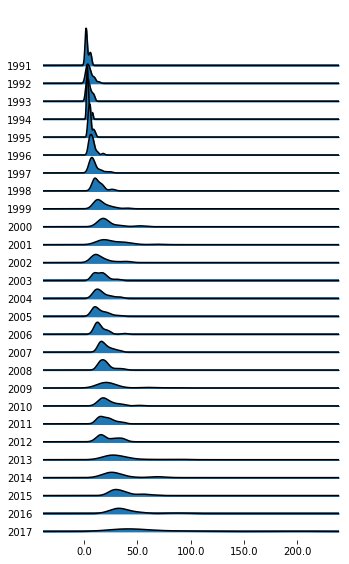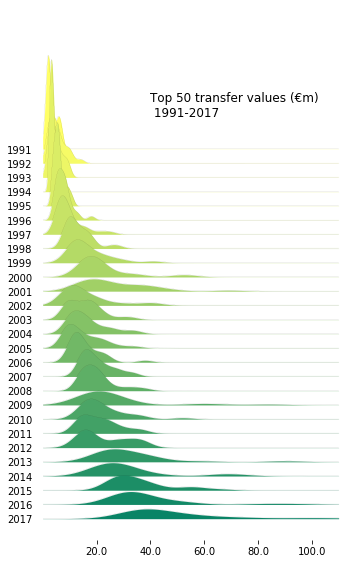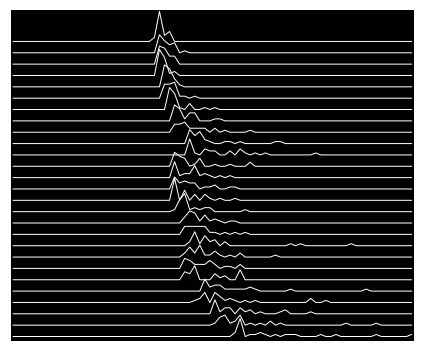# Joyplots in Python with Joypy

Joyplots are a way for us to show lots of density plots in one chart, while also adding a category that we can differentiate by. They are quite fashionable at present and have allowed for some beautiful graphics. Python’s joypy library, building on matplotlib, gives us the opportunity to create our very own joyplots in just a few lines of code. In this article, we’ll give a tutorial into creating the plots and customising them by plotting the top 50 transfer values of each year since 1991. Hopefully we’ll get a small insight into the trends of the biggest moves in the modern game.

Let’s get our modules in place and take a look at our dataset:

In :
```from __future__ import unicode_literals
import joypy
import pandas as pd
from matplotlib import pyplot as plt
from matplotlib import cm
%matplotlib inline

```
Out:
Player Value Year
0 David Platt 7 1991
1 Robert Prosinecki 7 1991
2 Thomas Häßler 6 1991
3 Jürgen Kohler 6 1991
4 Thomas Doll 6 1991

Our dataset has 1350 observations, each containing one of the most expensive 50 transfers for the years 1991 through 2017.

When we create a joyplot, we should specify what category we want to differentiate. In this case, we will categorise by the ‘Year’ column, and we use the ‘by’ argument in ‘joypy.joyplot()’ to do this.

We should also tell it which column we want the density plots to draw. Here, it will obviously be the ‘value’ column – passed on through the ‘column’ argument.

Let’s take a look at the default:

In :
```fig, axes = joypy.joyplot(df, by="Year", column="Value",figsize=(5,8))
plt.show()
```It’s a start! Certainly not the best-looking plot, but we’ve got something.

As you can see, each plot-line is a year, with the graphic showing the values. The plot appears needlessly wide due to Neymar’s transfer forcing us to draw beyond 200m€. Is there anything in football oil money won’t affect?!

What can we learn from the chart? Obviously, players have gotten more expensive as time goes on – you certainly don’t need a chart to tell you that. But we can also see that the variation between values in the top 50 of each year has become much more spread out – we even see some years where the trend hasn’t been growth.

I don’t doubt that there are better ways to plot this, but hopefully we can make something fairly good-looking to justify it.

Let’s through in some customisation:

In :
```fig, axes = joypy.joyplot(df[df.Player != 'Neymar'], by="Year", column="Value",figsize=(5,8),
linewidth=0.05,overlap=3,colormap=cm.summer_r,x_range=[0,110])

plt.text(40, 0.8, "Top 50 transfer values (€m) \n 1991-2017",fontsize=12)

plt.show()
```In this plot, we have used a subset of our dataset – excluding Neymar, to try and get a better handle of the rest of the shapes in the chart.

Let’s run through the other changes we’ve made:

• We’ve set a very thin line-width, allowing us to see the odd outlying transfer fee – notice Ronaldo in 2009, Zidane in 2001 or Sheare rin 1996.
• The plots overlap and are much more condensed. This makes for a smaller plot, and the overlapping plots are a bit more interesting to look at.
• A colourmap is applied, changing the colour for each year. As we have overlapped the plots, we need to set a colour difference to tell our years apart.
• We’ve set custom limits to the x axis, stopping the overspill from negative numbers

There are still some changes that we would like to make. We should really add axis titles, annotate some interesting transfers and so on – but this is a great start and illustrates how we can make joyplots quickly and easily.

It would be remiss of any Joyplot tutorial not to plot the Unknown Pleasures cover that inspires the plot name. Borrowing code from the docs, and using the data above, let’s give it a go:

In :
```fig, axes = joypy.joyplot(df[df.Player != 'Neymar'],by="Year", column="Value", ylabels=False, xlabels=False,
grid=False, fill=False, background='k', linecolor="w", linewidth=1, x_range=[-60,110],
legend=False, overlap=0.5, figsize=(6,5),kind="counts", bins=80)
```Interestingly, we can see outliers a lot easier with this style! I quite like the aesthetics, but it is maybe not as eye-catching as a data visualisation compared to the previous plot.

### Summary

This article has taken you through the steps of creating and editing your first joyplots. These overlapping density plots can make really beautiful charts that show a lot of information in a novel way. These charts use time to differentiate each row on the y axis, but you may also want to find a way to plot time along the x axis to show changes over time, rather than changes in density.

Next up, take a look at plotting heatmaps for correlation, or our other visualisation articles.